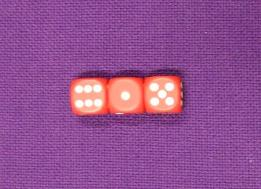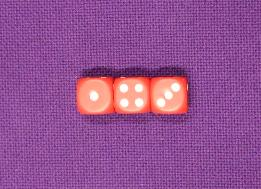#### You may also like### Pebbles

Place four pebbles on the sand in the form of a square. Keep adding as few pebbles as necessary to double the area. How many extra pebbles are added each time?### Bracelets

Investigate the different shaped bracelets you could make from 18 different spherical beads. How do they compare if you use 24 beads?### Sweets in a Box

How many different shaped boxes can you design for 36 sweets in one layer? Can you arrange the sweets so that no sweets of the same colour are next to each other in any direction?

# Three Dice

##### Age 7 to 11Challenge Level

Take a look at some ordinary dice.
What do you notice about the way the numbers are arranged?

Now look at these three dice in a row:The numbers on the tops of the dice read $6$, $1$ and $5$.

What do the numbers on the top add up to?

Can you use what you found out about the way the numbers are arranged to say what numbers are on the bottom of the dice? Were you correct?

What is the sum of the numbers on the bottoms of the dice?

Let's try that again.
This time the numbers on the top read $1$, $4$ and $3$.Can you work out the total?
Can you work out the numbers on the bottom and their total?

Try out some arrangements yourself. Each time record the sum of the numbers on the top and the sum of the numbers on the bottom.

Do you notice a relationship between the 'top sum' and the 'bottom sum'?
Can you explain it?

I experimented with arrangements where the top sum is a multiple of three, and find that in each case the bottom sum is also a multiple of three. Is it always true?

I try to arrange the dice so that the top and bottom sums are both multiples of four, but can't seem to be able to do it. Can you? Can you explain what you find out?

On the other hand, if I arrange four dice in a row it is easy to make the top and bottom sums both multiples of four. Can you arrange four dice so that the top and bottom sums are both multiples of three? Can you explain what you find out?